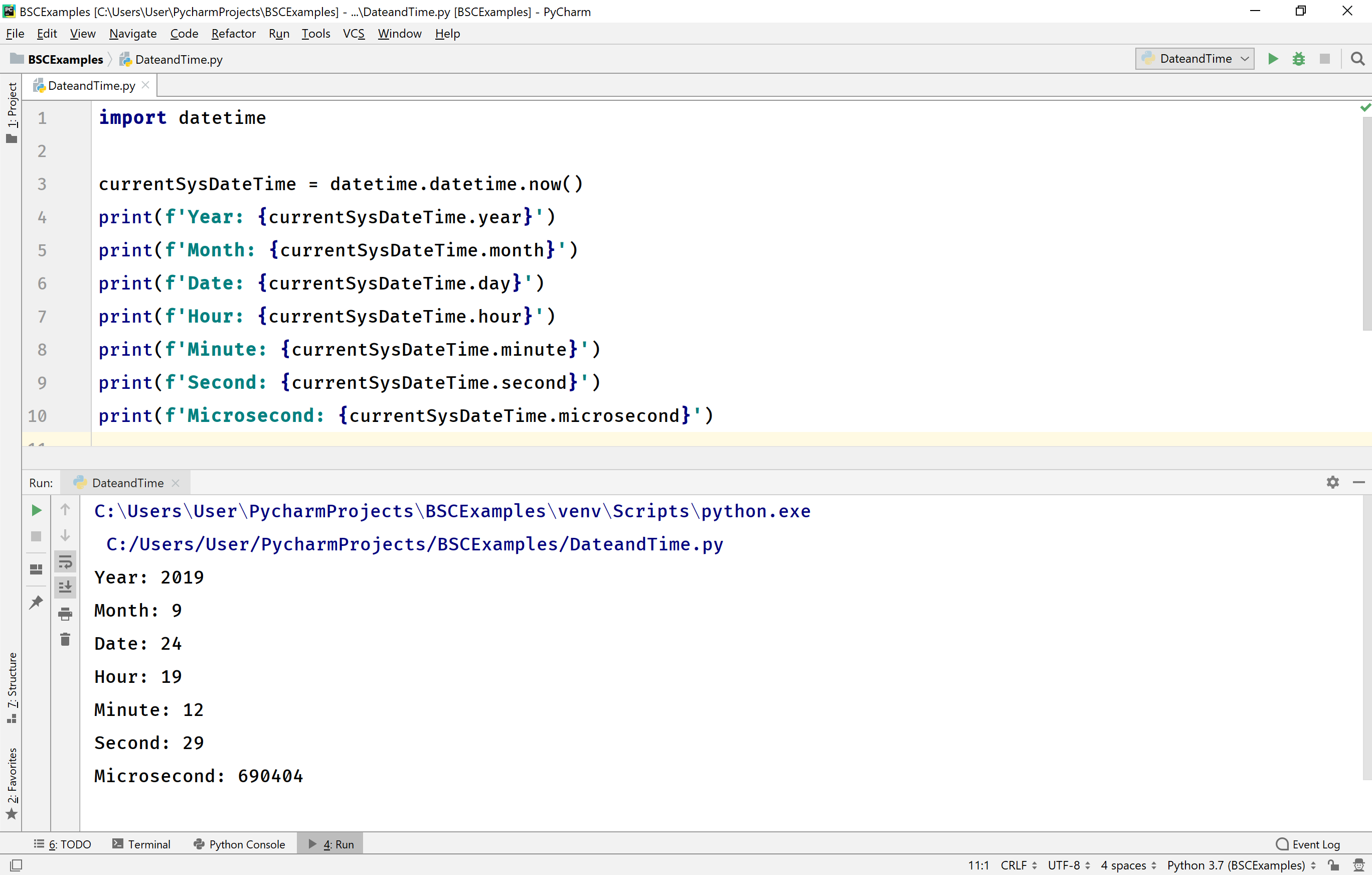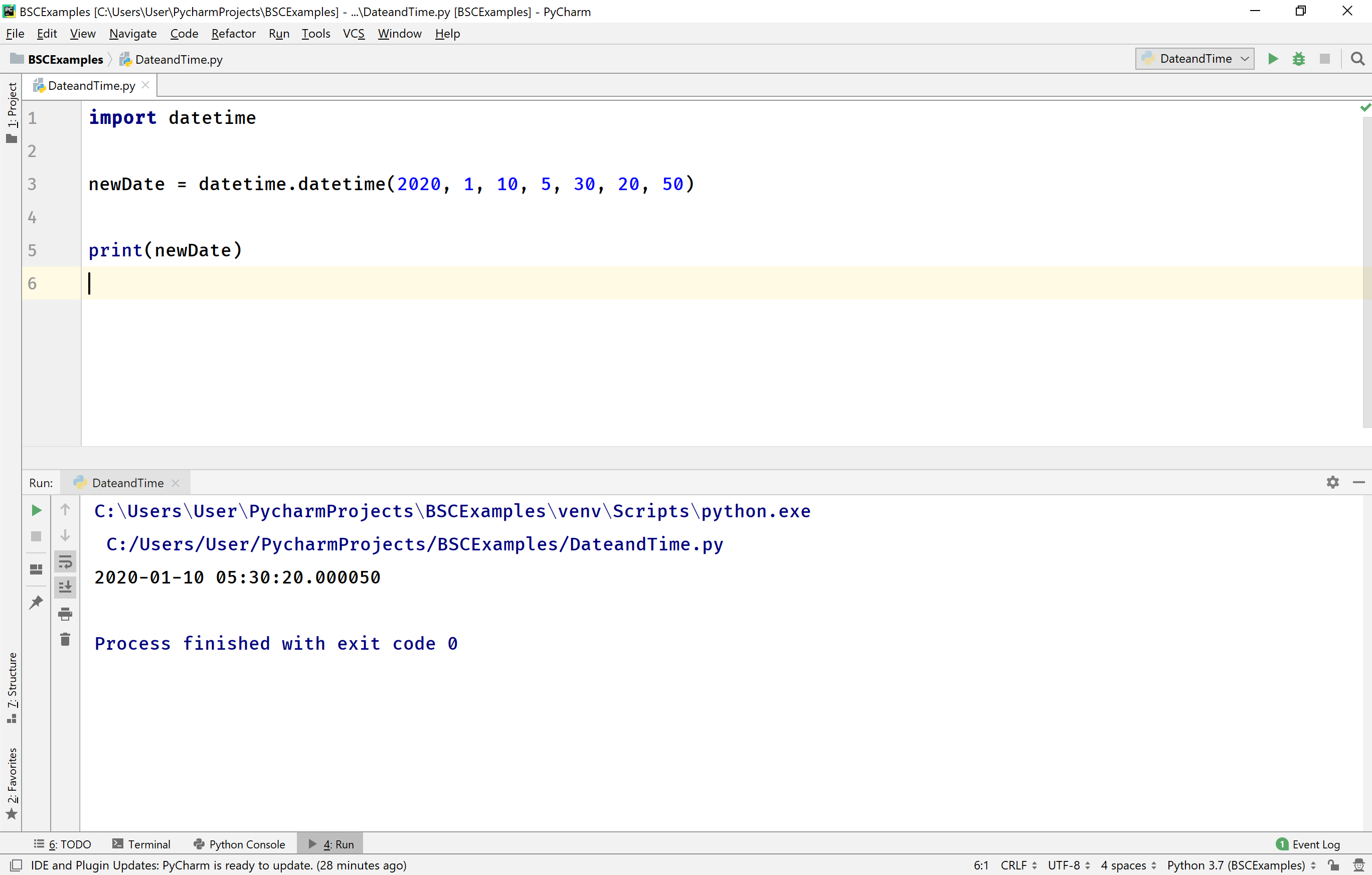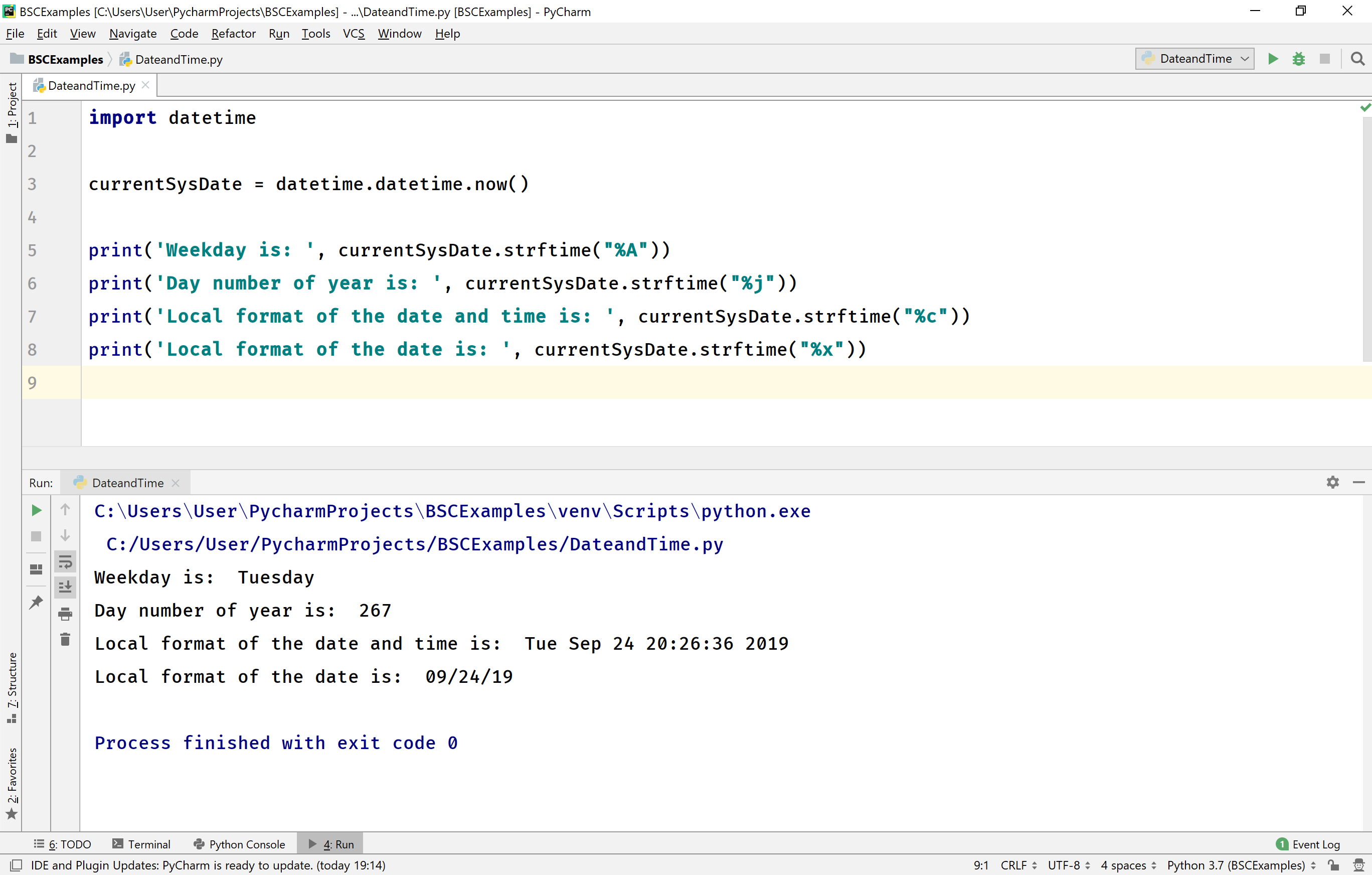The perfect place for easy learning...

×

# Python datetime

The Python programming language provides multiple built-in modules to work with date and time. The most popular and commonly used module is datetime. This module provides a wide range of built-in functions to work with date and time.

To use the built-in functions and objects that are provided by the datetime module, we must import this module using an import statement.

# Current System Date & Time

The datetime module provides a method now( ) to return current system data and time. It returns the date and time in the form of a datetime object. The datetime is not a data type, but its an object of dataetime class. Let's look at the following example code.

Example
``````import datetime

currentSysDateTime = datetime.datetime.now()

print(type(currentSysDateTime))
print(currentSysDateTime)
``````

When we run the above example code, it produces the following output.The datetime object contains the date in the form of YYYY-MM-DD HH:MM:Sec.Microsecond. That means, it contains Year with four digits, month with two digits, date with two digits, hour with two digits, minute with two digits, seconds with two digits, and microseconds six digits.

In Python, the datetime module has many built-in methods to work with date and time object values. Let's look at the following example code to use a few of them.

Example
``````import datetime

currentSysDateTime = datetime.datetime.now()

print(f'Year: {currentSysDateTime.year}')
print(f'Month: {currentSysDateTime.month}')
print(f'Date: {currentSysDateTime.day}')
print(f'Hour: {currentSysDateTime.hour}')
print(f'Minute: {currentSysDateTime.minute}')
print(f'Second: {currentSysDateTime.second}')
print(f'Microsecond: {currentSysDateTime.microsecond}')
``````

When we run the above example code, it produces the following output.# Creating datetime object

In Python, the datetime module allows us to create a datetime object with a specific date. To create a datetime object, we use the constructor of datetime class.

The datetime( ) constructor requires three parameters year, month (only 1 to 12), date (only 1 to 31). However, the range of date value depends on the month value. For example, for month 2 it allows only 1 to 29, for month 4 it allows only 1 to 30, etc.

Example
``````import datetime

newDate = datetime.datetime(2020, 5, 31)

print(newDate)
``````

The datetime( ) constructor creates the date with time 00:00:00 by default. But, optionally we may specify the hour, minute, seconds, and microsecond too.

Example
``````import datetime

newDate = datetime.datetime(2020, 1, 10, 5, 30, 20, 50)

print(newDate)
``````

When we run the above example code, it produces the following output.The datetime( ) constructor also allows to pass timezone which can be specified after the microseconds, and has a default value None.

# The strftime( ) built-in method

The datetime object provides a built-in method for formatting date object into a readable string. This built-in method requires only one parameter called format, and the datetime module provides the following formats.

Format Description Example
%Y Full Year 2019
%y Short Year 19
%B Full Month August
%b Short Month Aug
%m Month in numbers from 01 to 12 08
%d Day as number from 01 to 31 24
%A Full Weekday Tuesday
%a Short Weekday Tue
%w Weekday as number from 0 to 6 (0 is Sunday) 2
%H Hour as 24 hours format 20
%I Hour as 12 hours format 08
%p AM or PM PM
%M Minute from 00 to 59 10
%S Seconds from 00 to 59 45
%f Microsecond from 000000 to 999999 000055
%Z Timezone CST
%j Day number of year (001 to 366) 267
%U Week number of year (00 to 53) 38
%c Local Timezone format of date time Tue Sep 24 20:17:48 2019
%x Local format of Date 09/24/19
%X Local format of time 20:20:05

Let's look at the following example code.

Example
``````import datetime

currentSysDate = datetime.datetime.now()

print('Weekday is: ', currentSysDate.strftime("%A"))
print('Day number of year is: ', currentSysDate.strftime("%j"))
print('Local format of the date and time is: ', currentSysDate.strftime("%c"))
print('Local format of the date is: ', currentSysDate.strftime("%x"))
``````

When we run the above example code, it produces the following output.